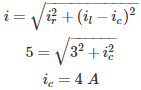# MCQs on Electric Circuits

##### Page 6 of 63. Go to page 1 2 3 4 5 6 7 8 9 10 11 12 13 14 15 16 17 18 19 20 21 22 23 24 25 26 27 28 29 30 31 32 33 34 35 36 37 38 39 40 41 42 43 44 45 46 47 48 49 50 51 52 53 54 55 56 57 58 59 60 61 62 63
01․ In RLC parallel circuit circuit current through inductor is more than current through capacitor. Then what is the power factor of the circuit?
lagging
unity
zero

In this circuit inductive effect is more than capacitive effect. Therefore power factor is lagging in nature.

02․ When power factor angle is constant, then shape of the current locus is
semi circle.
circle.
triangle.
straight line.

The path traced by the terminus of the current vector by varying either any one of the circuit element or by varying source frequency is called as current locus. When the power factor angle constant the shape of the locus is straight line.

03․ When power factor angle is varying, the shape of the current locus is
Circle
Semicircle
Triangle
Straight line

The shape of the current locus is the inverse of a straight line which is a circle passing through origin.

04․ Current locus is obtained by which of the following?
Varying any one of the circuit element
Varying source frequency
Either of these
None of the above

The path traced by the terminus of the current vector by varying either any one of the circuit element or by varying source frequency is called as current locus.

05․ In parallel RC circuit total current is 5A and current through resistor is 3A. What is the current through capacitor?
5A
2A
3A
4A06․ In RLC parallel circuit if current through capacitor and inductor is equal. Then What is the power factor?
lagging
unity
zero

In this type of circuit reactive power required by the inductor is supplied by the capacitor. Inductive reactance is balanced by capacitive reactance. Therefore the power factor of the network is unity.

07․ For occurrence of resonance which of the following elements are required?
R
L
C
Both 2 and 3

For occurrence of resonance, the impedance of any network should not have any imaginary part hence capacitive reactance should be cancelled by inductive reactance. Therefore both L and C are required. Then impedance is equal to resistance.

08․ Under resonance condition the phase angle between voltage phase and current phase is
90°
- 90°
45°

Under series or parallel resonance condition there would not be any reactive part, the circuit is completely resistive. Hence, voltage phase is in phase with the current phase. Therefore angle between voltage phase and current phase is zero degree.

09․ Voltage magnification will occur in which of the following case?
Series resonance
Parallel resonance
Both
None of the above

Voltage across inductor or voltage across capacitor is greater than the source voltage, this phenomenon is called voltage magnification. This will occur in series resonance. But at series resonance condition the voltage across the inductor cancel out the voltage across the capacitor.

10․ Current magnification will occur in which of the following case?
Series resonance
Parallel resonance
Both
None of the above

Current in the inductor or capacitor is greater than the source current, this phenomenon is called current magnification. This will occur in case of parallel resonance. But at parallel resonance the inductive current component balances out the capacitive current component.

<<<45678>>>